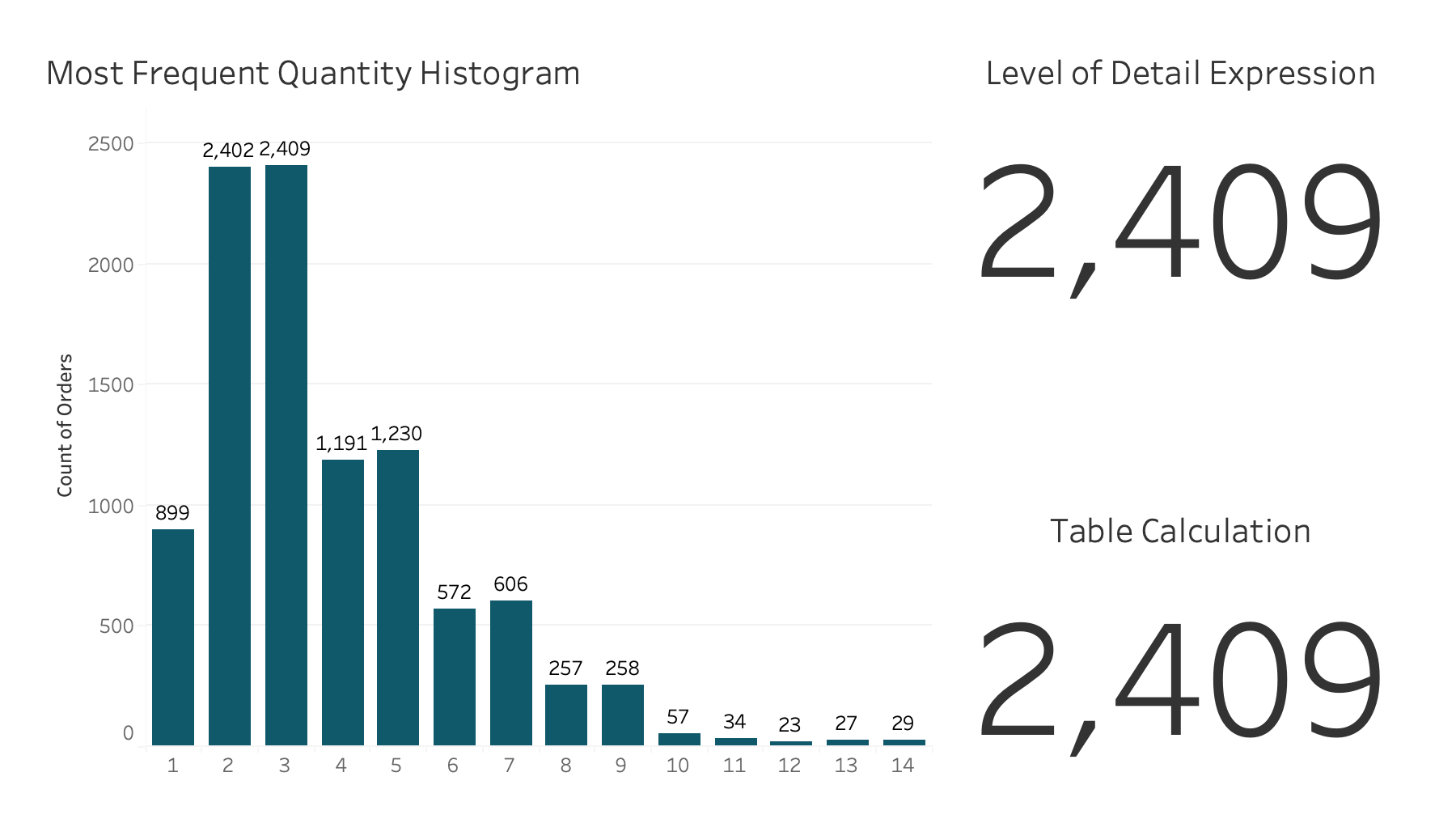Data Viz Done Right

# How to Calculate the Most Frequent Value of a MeasureIn this tip, I show you how to use both a level of detail expression and a table calculation to compute the most frequent value in a measure.

First, I show you how to return this as a histogram as well as a single number.

By the end of this tip you will be able to calculate the most frequent quantity ordered. This same process could be used, for example, to compute the most frequent discount.